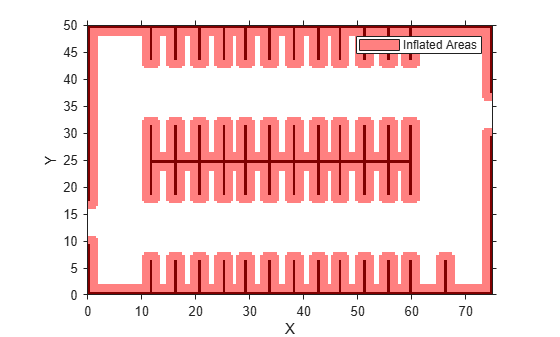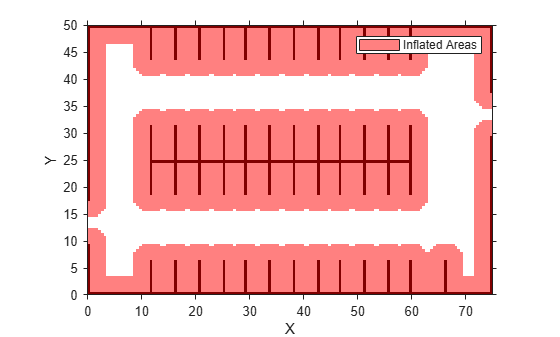Documentation

# pathPlannerRRT

Configure RRT* path planner

## Description

The `pathPlannerRRT` object configures a vehicle path planner based on the optimal rapidly exploring random tree (RRT*) algorithm. An RRT* path planner explores the environment around the vehicle by constructing a tree of random collision-free poses.

Once the `pathPlannerRRT` object is configured, use the `plan` function to plan a path from the start pose to the goal.

## Creation

### Syntax

``planner = pathPlannerRRT(costmap)``
``planner = pathPlannerRRT(costmap,Name,Value)``

### Description

example

````planner = pathPlannerRRT(costmap)` returns a `pathPlannerRRT` object for planning a vehicle path. `costmap` is a `vehicleCostmap` object specifying the environment around the vehicle. `costmap` sets the `Costmap` property value.```
````planner = pathPlannerRRT(costmap,Name,Value)` sets properties of the path planner by using one or more name-value pair arguments. For example, `pathPlanner(costmap,'GoalBias',0.5)` sets the `GoalBias` property to a probability of 0.5. Enclose each property name in quotes.```

## Properties

expand all

Costmap of the vehicle environment, specified as a `vehicleCostmap` object. The costmap is used for collision checking of the randomly generated poses. Specify this costmap when creating your `pathPlannerRRT` object using the `costmap` input.

Tolerance around the goal pose, specified as an [xTol, yTol, ΘTol] vector. The path planner finishes planning when the vehicle reaches the goal pose within these tolerances for the (x, y) position and the orientation angle, Θ. The xTol and yTol values are in the same world units as the `vehicleCostmap`. ΘTol is in degrees.

Probability of selecting the goal pose instead of a random pose, specified as a real scalar in the range [0, 1]. Large values accelerate reaching the goal at the risk of failing to circumnavigate obstacles.

Method used to calculate the connection between consecutive poses, specified as `'Dubins'` or `'Reeds-Shepp'`. Use `'Dubins'` if only forward motions are allowed.

The `'Dubins'` method contains a sequence of three primitive motions, each of which is one of these types:

• Straight (forward)

• Left turn at the maximum steering angle of the vehicle (forward)

• Right turn at the maximum steering angle of the vehicle (forward)

If you use this connection method, then the segments of the planned vehicle path are stored as an array of `driving.DubinsPathSegment` objects.

The `'Reeds-Shepp'` method contains a sequence of three to five primitive motions, each of which is one of these types:

• Straight (forward or reverse)

• Left turn at the maximum steering angle of the vehicle (forward or reverse)

• Right turn at the maximum steering angle of the vehicle (forward or reverse)

If you use this connection method, then the segments of the planned vehicle path are stored as an array of `driving.ReedsSheppPathSegment` objects.

The `MinTurningRadius` property determines the maximum steering angle.

Maximum distance between two connected poses, specified as a positive real scalar. `pathPlannerRRT` computes the connection distance along the path between the two poses, with turns included. Larger values result in longer path segments between poses.

Minimum turning radius of the vehicle, specified as a positive real scalar. This value corresponds to the radius of the turning circle at the maximum steering angle. Larger values limit the maximum steering angle for the path planner, and smaller values result in sharper turns. The default value is calculated using a wheelbase of 2.8 meters with a maximum steering angle of 35 degrees.

Minimum number of planner iterations for exploring the costmap, specified as a positive integer. Increasing this value increases the sampling of alternative paths in the costmap.

Maximum number of planner iterations for exploring the costmap, specified as a positive integer. Increasing this value increases the number of samples for finding a valid path. If a valid path is not found, the path planner exits after exceeding this maximum.

Enable approximate nearest neighbor search, specified as `true` or `false`. Set this value to `true` to use a faster, but approximate, search algorithm. Set this value to `false` to use an exact search algorithm at the cost of increased computation time.

## Object Functions

 `plan` Plan vehicle path using RRT* path planner `plot` Plot path planned by RRT* path planner

## Examples

collapse all

Plan a vehicle path to a parking spot by using the RRT* algorithm.

Load a costmap of a parking lot. Plot the costmap to see the parking lot and inflated areas for the vehicle to avoid.

```data = load('parkingLotCostmapReducedInflation.mat'); costmap = data.parkingLotCostmapReducedInflation; plot(costmap)```Define start and goal poses for the path planner as [x, y, Θ] vectors. World units for the (x,y) locations are in meters. World units for the Θ orientation values are in degrees.

```startPose = [11, 10, 0]; % [meters, meters, degrees] goalPose = [31.5, 17, 90];```

Create an RRT* path planner to plan a path from the start pose to the goal pose.

```planner = pathPlannerRRT(costmap); refPath = plan(planner,startPose,goalPose);```

Plot the planned path.

`plot(planner)`Plan a vehicle path through a parking lot by using the optimal rapidly exploring random tree (RRT*) algorithm. Check that the path is valid, and then plot the transition poses along the path.

Load a costmap of a parking lot. Plot the costmap to see the parking lot and inflated areas for the vehicle to avoid.

```data = load('parkingLotCostmap.mat'); costmap = data.parkingLotCostmap; plot(costmap)```Define start and goal poses for the vehicle as [x, y, Θ] vectors. World units for the (x,y) locations are in meters. World units for the Θ orientation angles are in degrees.

```startPose = [4, 4, 90]; % [meters, meters, degrees] goalPose = [30, 13, 0];```

Use a `pathPlannerRRT` object to plan a path from the start pose to the goal pose.

```planner = pathPlannerRRT(costmap); refPath = plan(planner,startPose,goalPose);```

Check that the path is valid.

`isPathValid = checkPathValidity(refPath,costmap)`
```isPathValid = logical 1 ```

Interpolate the transition poses along the path.

`transitionPoses = interpolate(refPath);`

Plot the planned path and the transition poses on the costmap.

```hold on plot(refPath,'DisplayName','Planned Path') scatter(transitionPoses(:,1),transitionPoses(:,2),[],'filled', ... 'DisplayName','Transition Poses') hold off```## Tips

• Updating any of the properties of the planner clears the planned path from `pathPlannerRRT`. Calling `plot` displays only the costmap until a path is planned using `plan`.

• To improve performance, the `pathPlannerRRT` object uses an approximate nearest neighbor search. This search technique checks only `sqrt(N)` nodes, where `N` is the number of nodes to search. To use exact nearest neighbor search, set the `ApproximateSearch` property to `false`.

• The Dubins and Reeds-Shepp connection methods are assumed to be kinematically feasible and ignore inertial effects. These methods make the path planner suitable for low velocity environments, where inertial effects of wheel forces are small.

 Karaman, Sertac, and Emilio Frazzoli. "Optimal Kinodynamic Motion Planning Using Incremental Sampling-Based Methods." 49th IEEE Conference on Decision and Control (CDC). 2010.

 Shkel, Andrei M., and Vladimir Lumelsky. "Classification of the Dubins Set." Robotics and Autonomous Systems. Vol. 34, Number 4, 2001, pp. 179–202.

 Reeds, J. A., and L. A. Shepp. "Optimal paths for a car that goes both forwards and backwards." Pacific Journal of Mathematics. Vol. 145, Number 2, 1990, pp. 367–393.